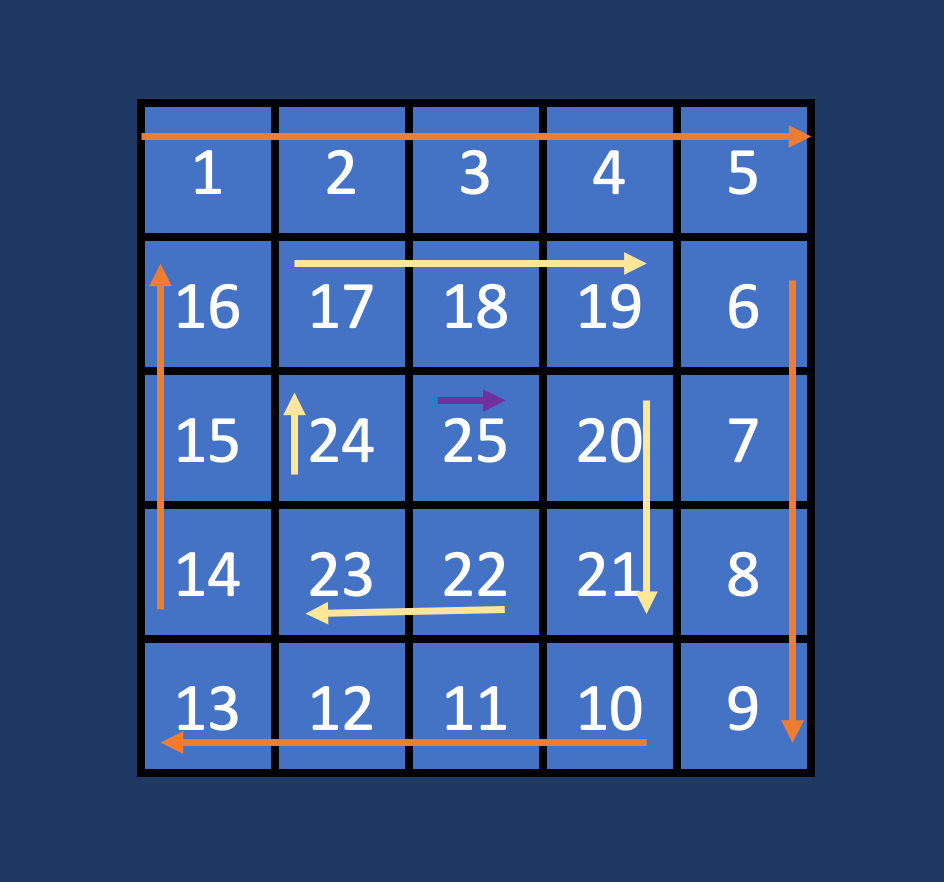# Leetcode 59 — Spiral Matrix 2

Today we’ll cover and explain the solution to Leetcode 59 — Spiral Matrix 2. A problem that gets asked by elites like Apple, Microsoft, and Amazon.

# Problem Overview

Given a positive integer `n`, generate an `n x n` matrix filled with elements from `1` to `n^2` in spiral order.

Constraints:

`1 <= n <= 20`

This problem description is straight forward, and the only ambiguity may come from the definition of spiral. Here, spiral implies a left to right, top to bottom, and then inward pattern. If this isn’t clear, the example below should reiterate the pattern.

# Example

We’re going to walk through an expanded example, over the leetcode example, so that we can see the pattern.

`Input: n = 5Output: [[1, 2, 3, 4, 5], [16, 17, 18, 19, 6], [15, 24, 25, 20, 7], [14, 23, 22, 21, 8], [13, 12, 11, 10, 9]]Explanation: (Depiction below)`In Orange is the outer spiral, in Yellow is the middle spiral, and in Purple is the final spiral.

Color coding the layer of the spiral reveals a pattern that is crucial to solving this problem. I’ll expand up the pattern in the next section.

# Solution Discussion

The first important concept to understand is that the spiral has layers. What is a layer? It is a ring in the spiral. Referring to the depiction above, layer `0` would be the outer most ring, or the ring traced with the orange arrows. Notice how with `n=5` there are 3 layers. What happens when `n=6`? If you draw it out we’ll see there are also 3 layers. One last question here, draw out `n=2`. You’ll find out you have 1 layer.

Hopefully you’ve noticed that the number of layers is equivalent to the follow equation

Next, let’s take a look at the coordinates:

Once you write out the coordinates, the solution starts to become obvious. We construct a for loop over the layers, and fill in each layer, incrementing a counting variable as our `n`. The tricky part is filling in each direction. In this solution, I’ve opted to use sub-loops to handle each direction, but the tricky part is determining the bounds. We don’t want to overwrite coordinates, so we can’t directly use the coordinates we’ve determined above, but CAN use them to determine the correct set to use. Take for example that outer layer:

Using the coordinates we initially determined, we can easily get the correct ones by subtracting one (in this case) or adding one, where appropriate. However, you’ll come to a question of how to fill in the left side, where our indices are decreasing. In my solution, I’ve opted to use the bounds we’ve determined, but start at the max index and iterate backwards (which is easily doable with some pythonic syntax).

Ultimately, we can put all this together and arrive at the following solution:

I hope you enjoyed the article :)))

--

--## Thomas Higginson

Software Engineer working on Cognitive EW capabilities, and human that enjoys making smiles. Welcome.